# Power Conversion Circuits

## Discrete Semiconductor Devices and Circuits

• #### Question 1

 Don’t just sit there! Build something!!

Learning to mathematically analyze circuits requires much study and practice. Typically, students practice by working through lots of sample problems and checking their answers against those provided by the textbook or the instructor. While this is good, there is a much better way.

You will learn much more by actually building and analyzing real circuits, letting your test equipment provide the “answers” instead of a book or another person. For successful circuit-building exercises, follow these steps:

1. Carefully measure and record all component values prior to circuit construction, choosing resistor values high enough to make damage to any active components unlikely.
2. Draw the schematic diagram for the circuit to be analyzed.
3. Carefully build this circuit on a breadboard or other convenient medium.
4. Check the accuracy of the circuit’s construction, following each wire to each connection point, and verifying these elements one-by-one on the diagram.
5. Mathematically analyze the circuit, solving for all voltage and current values.
6. Carefully measure all voltages and currents, to verify the accuracy of your analysis.
7. If there are any substantial errors (greater than a few percent), carefully check your circuit’s construction against the diagram, then carefully re-calculate the values and re-measure.

When students are first learning about semiconductor devices, and are most likely to damage them by making improper connections in their circuits, I recommend they experiment with large, high-wattage components (1N4001 rectifying diodes, TO-220 or TO-3 case power transistors, etc.), and using dry-cell battery power sources rather than a benchtop power supply. This decreases the likelihood of component damage.

As usual, avoid very high and very low resistor values, to avoid measurement errors caused by meter “loading” (on the high end) and to avoid transistor burnout (on the low end). I recommend resistors between 1 kΩ and 100 kΩ.

One way you can save time and reduce the possibility of error is to begin with a very simple circuit and incrementally add components to increase its complexity after each analysis, rather than building a whole new circuit for each practice problem. Another time-saving technique is to re-use the same components in a variety of different circuit configurations. This way, you won’t have to measure any component’s value more than once.

• #### Question 2

Describe what a dynamotor is, and what its purpose might be in an electrical system.

• #### Question 3

What is a DC-DC converter circuit, and what applications might such a circuit be used for?

• #### Question 4

This circuit uses an 8038 waveform generator IC (integrated circuit) to produce a “sawtooth” waveform, which is then compared against a variable DC voltage from a potentiometer: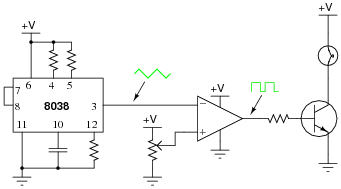The result is a pulse waveform to the base of the power transistor, of the same frequency as the sawtooth waveform. Normally in circuits such as this, the frequency is at least several hundred Hertz.

Explain what happens to the brightness of the lamp when the potentiometer wiper is moved closer to V, and when it is moved closer to ground.

• #### Question 5

This circuit generates a pulse of DC voltage sufficient to energize the neon lamp, every time the switch is opened: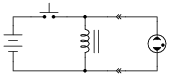Describe the principle of operation for this simple circuit, and also how it could be modified to produce continuous high-voltage DC power.

Hint: how does a common AC-DC power supply circuit convert pulses of rectified DC into a relatively “smooth” DC output?

• #### Question 6

The schematic diagram shown here is for a “buck” converter circuit, a type of DC-DC “switching” power conversion circuit: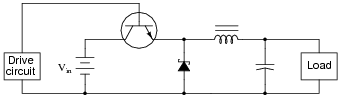In this circuit, the transistor is either fully on or fully off; that is, driven between the extremes of saturation or cutoff. By avoiding the transistor’s “active” mode (where it would drop substantial voltage while conducting current), very low transistor power dissipations can be achieved. With little power wasted in the form of heat, “switching” power conversion circuits are typically very efficient.

Trace all current directions during both states of the transistor. Also, mark the inductor’s voltage polarity during both states of the transistor.

• #### Question 7

The schematic diagram shown here is for a “boost” converter circuit, a type of DC-DC “switching” power conversion circuit: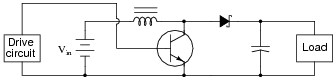In this circuit, the transistor is either fully on or fully off; that is, driven between the extremes of saturation or cutoff. By avoiding the transistor’s “active” mode (where it would drop substantial voltage while conducting current), very low transistor power dissipations can be achieved. With little power wasted in the form of heat, “switching” power conversion circuits are typically very efficient.

Trace all current directions during both states of the transistor. Also, mark the inductor’s voltage polarity during both states of the transistor.

• #### Question 8

The schematic diagram shown here is for an “inverting” converter circuit, a type of DC-DC “switching” power conversion circuit: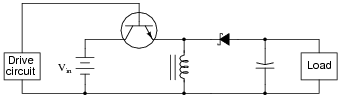In this circuit, the transistor is either fully on or fully off; that is, driven between the extremes of saturation or cutoff. By avoiding the transistor’s “active” mode (where it would drop substantial voltage while conducting current), very low transistor power dissipations can be achieved. With little power wasted in the form of heat, switching” power conversion circuits are typically very efficient.

Trace all current directions during both states of the transistor. Also, mark the inductor’s voltage polarity during both states of the transistor.

• #### Question 9

The schematic diagram shown here is for a “Cuk” converter circuit, a type of DC-DC “switching” power conversion circuit: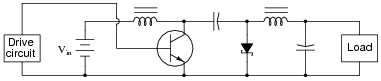In this circuit, the transistor is either fully on or fully off; that is, driven between the extremes of saturation or cutoff. By avoiding the transistor’s “active” mode (where it would drop substantial voltage while conducting current), very low transistor power dissipations can be achieved. With little power wasted in the form of heat, “switching” power conversion circuits are typically very efficient.

Trace all current directions during both states of the transistor. Also, mark the both inductors’ voltage polarities during both states of the transistor.

• #### Question 10

Predict how the operation of this buck converter circuit will be affected as a result of the following faults. Consider each fault independently (i.e. one at a time, no multiple faults):Drive circuit fails with a constant “low” (0 volts) output signal:
Drive circuit fails with a constant “high” ( V) output signal:
Diode fails shorted:
Inductor fails open:
Capacitor fails shorted:

For each of these conditions, explain why the resulting effects will occur.

• #### Question 11

Predict how the operation of this boost converter circuit will be affected as a result of the following faults. Consider each fault independently (i.e. one at a time, no multiple faults):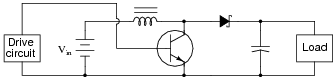Drive circuit fails with a constant “low” (0 volts) output signal:
Drive circuit fails with a constant “high” ( V) output signal:
Diode fails shorted:
Inductor fails open:
Capacitor fails shorted:

For each of these conditions, explain why the resulting effects will occur.

• #### Question 12

So-called linear regulator circuits work by adjusting either a series resistance or a shunt resistance to maintain output voltage at some fractional value of input voltage: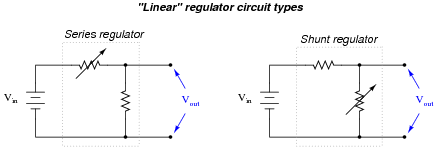Typically, these variable resistances are provided by transistors rather than actual rheostats, which would have to be manually controlled.

Explain why a switching regulator circuit would perform the same task as a linear regulator circuit at a much greater efficiency. Also, identify which type(s) of switching regulator circuit would be best suited for the task of reducing an input voltage to a lesser output voltage.

• #### Question 13

Shown here are two voltage-reducing circuits: both reducing a supply voltage of 13.5 volts down to 5 volts for a load.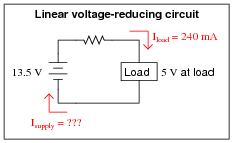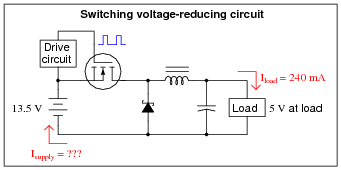Calculate the average supply current (Isupply) for both of these circuits. Assume that the switching circuit has negligible power losses in the transistor, inductor, capacitor, and diode. If the 13.5 volt source were an electrochemical battery, which battery would last longer powering the same load?

• #### Question 14

The output voltage of a buck converter circuit is a function of the input voltage and the duty cycle of the switching signal, represented by the variable D (ranging in value from 0% to 100%), where $$D=\frac{t_{on}}{t_{on}+t_{off}}$$: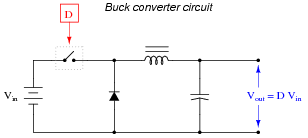Based on this mathematical relationship, calculate the output voltage of this converter circuit at these duty cycles, assuming an input voltage of 40 volts:

D = 0% ; Vout =
D = 25% ; Vout =
D = 50% ; Vout =
D = 75% ; Vout =
D = 100% ; Vout =
• #### Question 15

The output voltage of a boost converter circuit is a function of the input voltage and the duty cycle of the switching signal, represented by the variable D (ranging in value from 0% to 100%), where D = [(ton)/(ton toff)]: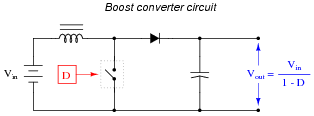Based on this mathematical relationship, calculate the output voltage of this converter circuit at these duty cycles, assuming an input voltage of 40 volts:

D = 0% ; Vout =
D = 25% ; Vout =
D = 50% ; Vout =
D = 75% ; Vout =
D = 100% ; Vout =
• #### Question 16

The output voltage of an inverting converter circuit is a function of the input voltage and the duty cycle of the switching signal, represented by the variable D (ranging in value from 0% to 100%), where $$D = \frac{t_{on}}{t_{on}+t_{off}}$$: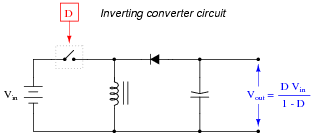Based on this mathematical relationship, calculate the output voltage of this converter circuit at these duty cycles, assuming an input voltage of 40 volts:

D = 0% ; Vout =
D = 25% ; Vout =
D = 50% ; Vout =
D = 75% ; Vout =
D = 100% ; Vout =
• #### Question 17

The output voltage of a Cuk converter circuit (named after the engineer who invented it) is a function of the input voltage and the duty cycle of the switching signal, represented by the variable D (ranging in value from 0% to 100%), where $$D = \frac{t_{on}}{t_{on}+t_{off}}$$: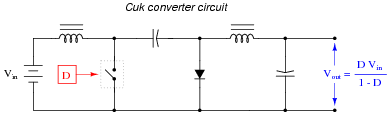Based on this mathematical relationship, calculate the output voltage of this converter circuit at these duty cycles, assuming an input voltage of 25 volts:

D = 0% ; Vout =
D = 25% ; Vout =
D = 50% ; Vout =
D = 75% ; Vout =
D = 100% ; Vout =
• #### Question 18

The following equations solve for the output voltage of various switching converter circuits (unloaded), given the switch duty cycle D and the input voltage:

$$V_{out}=DV_{in} \ \ \ \ \ \ \ \ \ \ (Buck \ \ converter \ \ circuit)$$

$$V_{out}=\frac{V_{in}}{1-D} \ \ \ \ \ \ \ \ \ \ (Boost \ \ converter \ \ circuit)$$

$$V_{out}=\frac{DV_{in}}{1-D} \ \ \ \ \ \ \ \ \ \ (Inverting \ \ or \ \ Cuk \ \ converter \ \ circuit)$$

Manipulate each of these equations to solve for duty cycle (D) in terms of the input voltage (Vin) and desired output voltage (Vout). Remember that duty cycle is always a quantity between 0 and 1, inclusive.

• #### Question 19

Many switching converter circuits use a switched MOSFET in place of a free-wheeling diode, like this: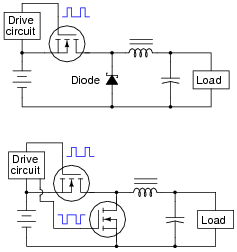The diode is a simple solution for providing the inductor a path for current when the main switching transistor is off. Why would anyone use another MOSFET in place of it, especially if this means the drive circuit has to become more complex (to drive two transistors at different times instead of just one transistor) to do the same task?

• #### Question 20

A “boost” switching converter operating at 90% efficiency delivers 50 volts to a DC load. Calculate the load current if the input voltage is 17 volts and the input current is 9.3 amps.

• #### Question 21

A “buck” switching converter operating at 85% efficiency delivers 10 amps of current at 5 volts to a DC load. Calculate the input current if the input voltage is 23 volts.

• #### Question 22

A “boost” switching converter operating at 80% efficiency delivers 178 volts at 1 amp to a DC load. Calculate the input voltage if the input current is 11 amps.

• #### Question 23

The output voltage of a buck converter is a direct function of the switching transistor’s duty cycle. Specifically, $$V_{out} = V_{in}(\frac{t_{on}}{t_{total}})$$. Explain how the following PWM control circuit regulates the output voltage of the buck converter: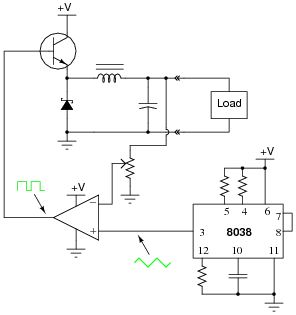• #### Question 24

The energy efficiency (η) of switching converter circuits typically remains fairly constant over a wide range of voltage conversion ratios. Describe how a switching regulator circuit (controlling load voltage to a pre-set value) “appears” to a power source of changing voltage if the regulator’s load is constant. In other words, as the input voltage changes, what does the input current do?

• #### Question 25

The following DC-DC converter circuit is called a forward converter. It is called this because the energy transfer from input to output occurs while the transistor is conducting, not while it is off. Verify this feature of the circuit by tracing current through all portions of it while the transistor is on: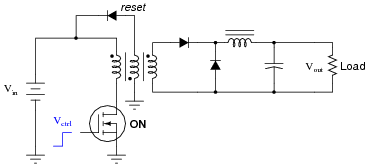Now, trace current through the circuit while the transistor is off, and explain the purpose of the reset winding in the transformer: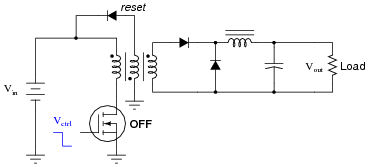• #### Question 26

While simple “brute-force” AC-DC power supply circuits (transformer, rectifier, filter, regulator) are still used in a variety of electronic equipment, another form of power supply is more prevalent in systems where small size and efficiency are design requirements. This type of power supply is called a switching power supply.

Explain what a “switching power supply” is, and provide a schematic diagram of one for presentation and discussion. (Hint: most electronic computers use “switching” power supplies instead of “brute force” power supplies, so schematic diagrams should not be difficult to find.)

• #### Question 27

Suppose a friend of yours recently purchased an off-road vehicle. This friend also purchased a military-surplus spotlight, which he thinks would be a great accessory for off-road illumination at night. The only problem is, the spotlight is rated for 24 volts, while the electrical system in his vehicle is 12 volt.

Your friend asks you to engineer a solution for powering the 24-volt spotlight with the 12 volts available on his vehicle. Of course, you are not allowed to modify the vehicle’s electrical system (change it to 24 volt generator, battery, starter motor, etc.), because it is new and still under warranty. What do you recommend to your friend?

Draw a component-level schematic diagram of your solution to this problem.

• #### Question 28

Describe the purpose and function of this circuit: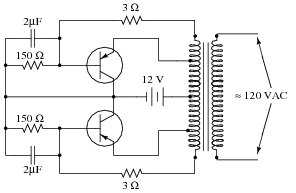The 120 volt AC output provided by this circuit is definitely not sinusoidal, and the circuit’s frequency varies with load. Can you think of any way(s) to improve these aspects of the circuit (you need not show details of your design modifications)?

• #### Question 29

Electronic power conversion circuits known as inverters convert DC into AC by using transistor switching elements to periodically reverse the polarity of the DC voltage. Usually, inverters also increase the voltage level of the input power by applying the switched-DC voltage to the primary winding of a step-up transformer. You may think of an inverter’s switching electronics as akin to double-pole, double-throw switch being flipped back and forth many times per second:The first commercially available inverters produced simple square-wave output: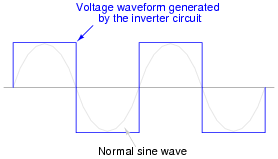However, this caused problems for most power transformers designed to operate on sine-wave AC power. When powered by the square-wave output of such an inverter, most transformers would saturate due to excessive magnetic flux accumulating in the core at certain points of the waveform’s cycle. To describe this in the simplest terms, a square wave possesses a greater volt-second product than a sine wave with the same peak amplitude and fundamental frequency.

This problem could be avoided by decreasing the peak voltage of the square wave, but then some types of powered equipment would experience difficulty due to insufficient (maximum) voltage: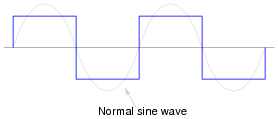A workable solution to this dilemma turned out to be a modified duty cycle for the square wave: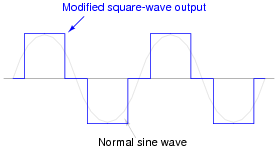Calculate the fraction of the half-cycle for which this modified square wave is “on,” in order to have the same volt-second product as a sine wave for one-half cycle (from 0 to π radians):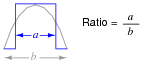Hint: it is a matter of calculating the respective areas underneath each waveform in the half-cycle domain.

• #### Question 30

A common topology for DC-AC power converter circuits uses a pair of transistors to switch DC current through the center-tapped winding of a step-up transformer, like this: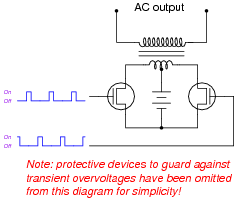In order for this form of circuit to function properly, the transistor “firing” signals must be precisely synchronized to ensure the two are never turned on simultaneously. The following schematic diagram shows a circuit to generate the necessary signals:Explain how this circuit works, and identify the locations of the frequency control and pulse duty-cycle control potentiometers.